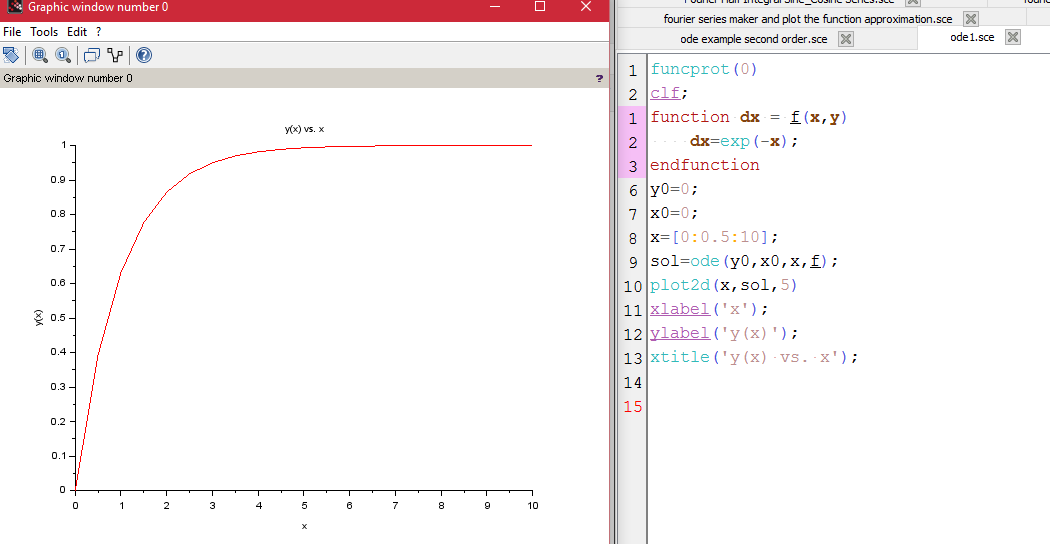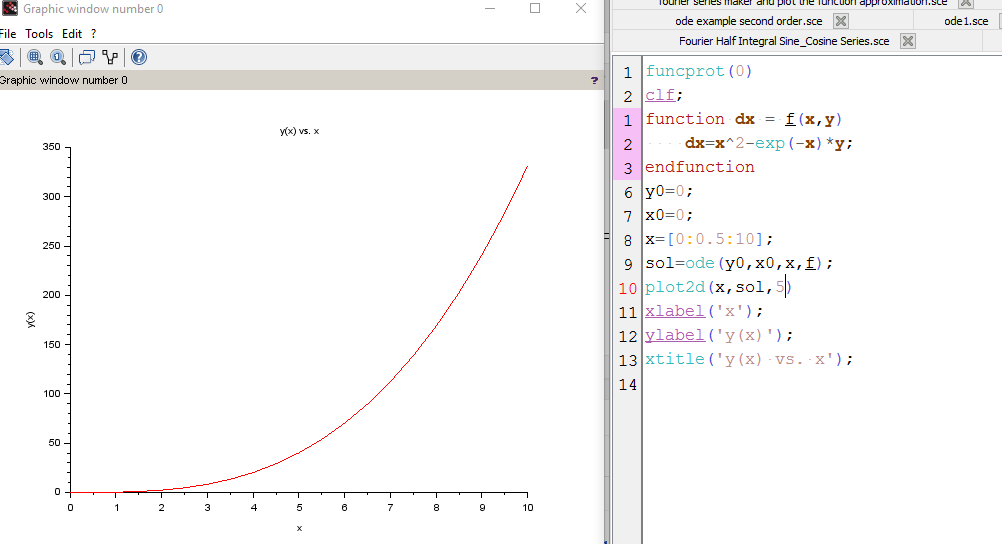# First Order Linear Differential Equations (ODE) in SCILAB

Scilab has a very important and useful in-built function ode() which can be used to evaluate an ordinary differential equation or a set of coupled first order differential equations.

The syntax is as follows:

y=ode(y0,x0,x,f)
where,
y0=initial value of y
x0=initial value of xx=value of x at which you want to calculate y.

The following examples illustrate the use of the ode to solve a given differential equation:

```funcprot(0)
clf;
function dx=f(x, y)
dx=exp(-x);
endfunction
y0=0;
x0=0;
x=[0:0.5:10];
sol=ode(y0,x0,x,f);
plot2d(x,sol,5)
xlabel('x');
ylabel('y(x)');
xtitle('y(x) vs. x');```

Output:```funcprot(0)
clf;
function dx=f(x, y)
dx=x^2-exp(-x)*y;
endfunction
y0=0;
x0=0;
x=[0:0.5:10];
sol=ode(y0,x0,x,f);
plot2d(x,sol,5)
xlabel('x');
ylabel('y(x)');
xtitle('y(x) vs. x');```

Output:Video:

If you’re new to Scilab and are looking for some good quality tutorials then check out this playlist:[wpedon id="7041" align="center"]

## 2 thoughts on “First Order Linear Differential Equations (ODE) in SCILAB”

1. can we solve harmonic oscillator using ode command?but i am facing some problem.can you solve any 2nd order differential equation(physics based)

1. Hi there,
I have in fact created a couple of tutorials on how to sole a second order ode. Scilab can solve it numerically and then you can plot the results. So I believe a harmonic oscillator should be easy simulate.